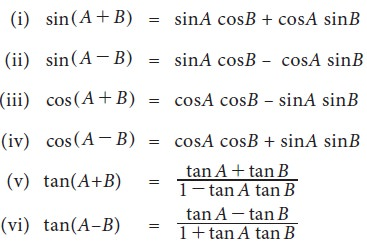Home | | Business Maths 11th std | Trigonometric ratios of compound angles

# Trigonometric ratios of compound angles

When we add or subtract angles, the result is called a compound angle.

Trigonometric ratios of compound angles

## 1. Compound angles

When we add or subtract angles, the result is called a compound angle. i.e.,the algebraic sum of two or more angles are called compound angles

For example, If A, B, C are three angles then A ± B, A + B + C, A – B + C etc., are compound angles.

## 2. Sum and difference formulae of sine, cosine and tangent## 3. Trigonometric ratios of multiple anglesTags : Definition, Formula, Solved Example Problems, Exercise | Mathematics , 11th Business Mathematics and Statistics(EMS) : Chapter 4 : Trigonometry
Study Material, Lecturing Notes, Assignment, Reference, Wiki description explanation, brief detail
11th Business Mathematics and Statistics(EMS) : Chapter 4 : Trigonometry : Trigonometric ratios of compound angles | Definition, Formula, Solved Example Problems, Exercise | Mathematics